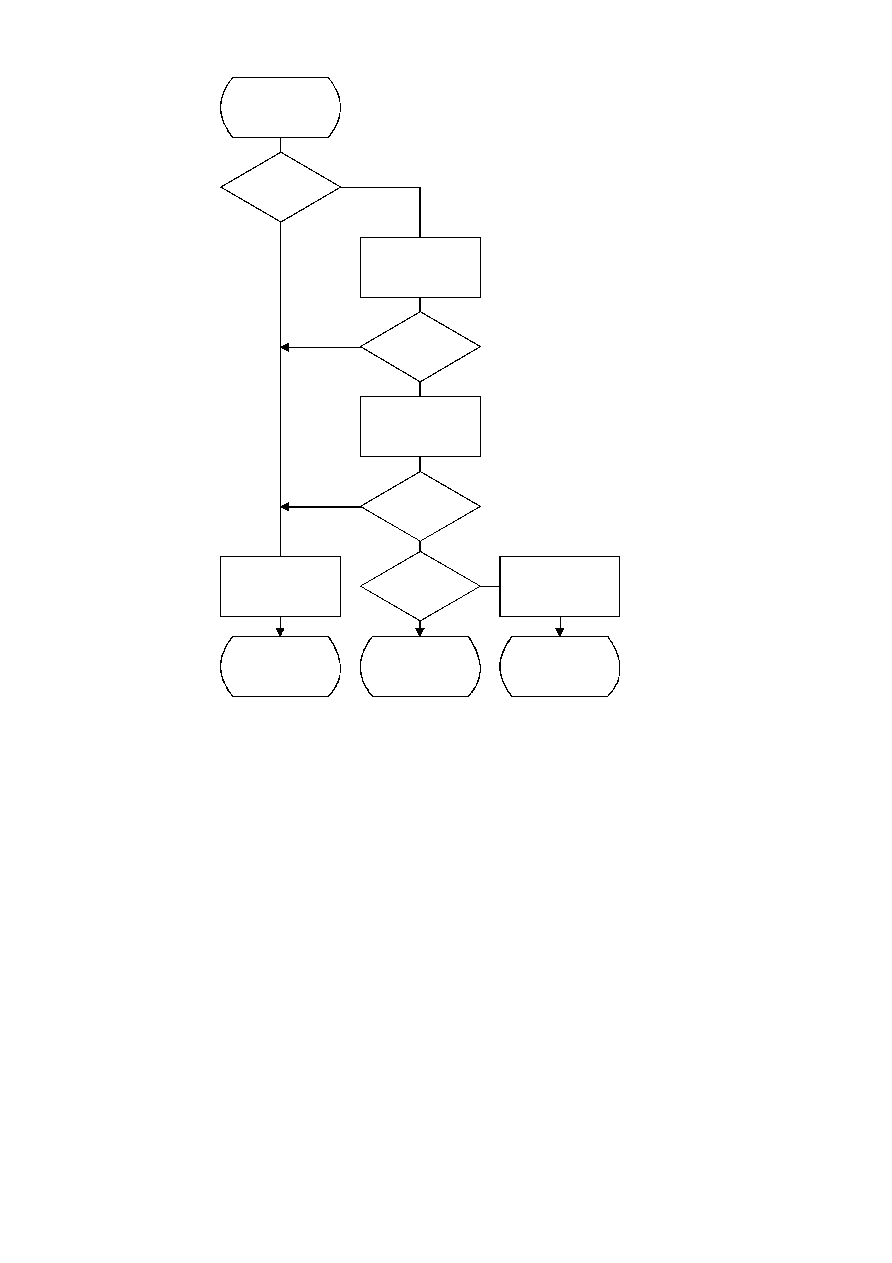ISO/IEC 10918-1 : 1993(E)
TISO1500-93/d088
NEXTBIT
CNT = 0
?
Yes
No
B = NEXTBYTE
CNT = 8
B = X'FF'
?
Yes
No
B2 = NEXTBYTE
B2 = 0
?
Yes
Yes
No
No
B2 = DNL
?
BIT = SRL B 7
CNT = CNT ­ 1
B = SLL B 1
Process DNL marker
Return BIT
Error
Terminate scan
Figure F.18 ­ Procedure for fetching the next bit of compressed data
Figure F.18 [D88] = 17 cm = 665 %
F.2.3
Sequential DCT decoding process with 8-bit precision extended to four sets of Huffman tables
This process is identical to the Baseline decoding process described in F.2.2, with the exception that the decoder shall be
capable of using up to four DC and four AC Huffman tables within one scan. Four DC and four AC Huffman tables is the
maximum allowed by this Specification.
F.2.4
Sequential DCT decoding process with arithmetic coding
This subclause describes the sequential DCT decoding process with arithmetic decoding.
The arithmetic decoding procedures for decoding binary decisions, initializing the statistical model, initializing the
decoder, and resynchronizing the decoder are listed in Table D.4 of Annex D.
Some of the procedures in Table D.4 are used in the higher level control structure for scans and restart intervals described
in F.2. At the beginning of scans and restart intervals, the probability estimates used in the arithmetic decoder are reset to
the standard initial value as part of the Initdec procedure which restarts the arithmetic coder.
CCITT Rec. T.81 (1992 E)
111